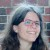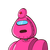# Find the value of k in x+y-5 and 3x+ky =7 which have no solution

Find the value of k in x+y-5 and 3x+ky =7 which have no solution

### 2 thoughts on “Find the value of k in x+y-5 and 3x+ky =7 which have no solution”

1.Step-by-step explanation:

### hence if k=3 then there will be no solution to this equations.

2.